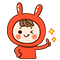2020/11/27 07:20

# array_sum () 计算数组中所有值的和。

number array_sum ( array $array ) 说明： array_sum() 将数组中的所有值的和以整数或浮点数的结果返回。 参数描述 array 输入的数组。 返回值： 所有值的和以整数或浮点数的结果返回。 实例：  <?php$a

=

array

(

2

,

4

,

6

,

8

)

;

echo

"sum(a) = "

.

array_sum

(

$a ) . "\n" ;$b

=

array

(

"a"

=

>

1.2

,

"b"

=

>

2.3

,

"c"

=

>

3.4

)

;

echo

"sum(b) = "

.

array_sum

(

\$b

)

.

"\n"

;

?

>





sum

(

a

)

=

20

sum

(

b

)

=

6.90
0 收藏

### 作者的其它热门文章0 评论
0 收藏
0